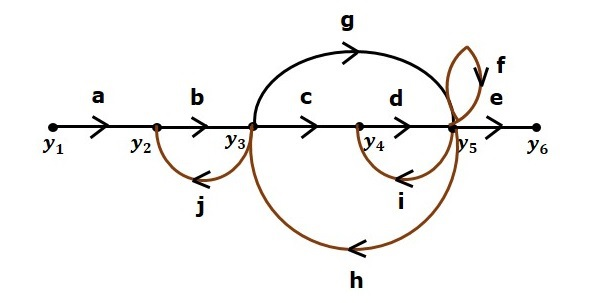# Mason's Gain Formula

Let us now discuss the Mason’s Gain Formula. Suppose there are ‘N’ forward paths in a signal flow graph. The gain between the input and the output nodes of a signal flow graph is nothing but the transfer function of the system. It can be calculated by using Mason’s gain formula.

Mason’s gain formula is

$$T=\frac{C(s)}{R(s)}=\frac{\Sigma ^N _{i=1}P_i\Delta _i}{\Delta}$$

Where,

• C(s) is the output node

• R(s) is the input node

• T is the transfer function or gain between $R(s)$ and $C(s)$

• Pi is the ith forward path gain

$\Delta =1-(sum \: of \: all \: individual \: loop \: gains)$

$+(sum \: of \: gain \: products \: of \: all \: possible \: two \:nontouching \: loops)$

$$-(sum \: of \: gain \: products \: of \: all \: possible \: three \: nontouching \: loops)+...$$

Δi is obtained from Δ by removing the loops which are touching the ith forward path.

Consider the following signal flow graph in order to understand the basic terminology involved here.### Path

It is a traversal of branches from one node to any other node in the direction of branch arrows. It should not traverse any node more than once.

Examples − $y_2 \rightarrow y_3 \rightarrow y_4 \rightarrow y_5$ and $y_5 \rightarrow y_3 \rightarrow y_2$

### Forward Path

The path that exists from the input node to the output node is known as forward path.

Examples − $y_1 \rightarrow y_2 \rightarrow y_3 \rightarrow y_4 \rightarrow y_5 \rightarrow y_6$ and $y_1 \rightarrow y_2 \rightarrow y_3 \rightarrow y_5 \rightarrow y_6$.

### Forward Path Gain

It is obtained by calculating the product of all branch gains of the forward path.

Examples − $abcde$ is the forward path gain of $y_1 \rightarrow y_2 \rightarrow y_3 \rightarrow y_4 \rightarrow y_5 \rightarrow y_6$ and abge is the forward path gain of $y_1 \rightarrow y_2 \rightarrow y_3 \rightarrow y_5 \rightarrow y_6$.

### Loop

The path that starts from one node and ends at the same node is known as loop. Hence, it is a closed path.

Examples − $y_2 \rightarrow y_3 \rightarrow y_2$ and $y_3 \rightarrow y_5 \rightarrow y_3$.

### Loop Gain

It is obtained by calculating the product of all branch gains of a loop.

Examples − $b_j$ is the loop gain of $y_2 \rightarrow y_3 \rightarrow y_2$ and $g_h$ is the loop gain of $y_3 \rightarrow y_5 \rightarrow y_3$.

### Non-touching Loops

These are the loops, which should not have any common node.

Examples − The loops, $y_2 \rightarrow y_3 \rightarrow y_2$ and $y_4 \rightarrow y_5 \rightarrow y_4$ are non-touching.

## Calculation of Transfer Function using Mason’s Gain Formula

Let us consider the same signal flow graph for finding transfer function.• Number of forward paths, N = 2.

• First forward path is - $y_1 \rightarrow y_2 \rightarrow y_3 \rightarrow y_4 \rightarrow y_5 \rightarrow y_6$.

• First forward path gain, $p_1 = abcde$.

• Second forward path is - $y_1 \rightarrow y_2 \rightarrow y_3 \rightarrow y_5 \rightarrow y_6$.

• Second forward path gain, $p_2 = abge$.

• Number of individual loops, L = 5.

• Loops are - $y_2 \rightarrow y_3 \rightarrow y_2$, $y_3 \rightarrow y_5 \rightarrow y_3$, $y_3 \rightarrow y_4 \rightarrow y_5 \rightarrow y_3$, $y_4 \rightarrow y_5 \rightarrow y_4$ and $y_5 \rightarrow y_5$.

• Loop gains are - $l_1 = bj$, $l_2 = gh$, $l_3 = cdh$, $l_4 = di$ and $l_5 = f$.

• Number of two non-touching loops = 2.

• First non-touching loops pair is - $y_2 \rightarrow y_3 \rightarrow y_2$, $y_4 \rightarrow y_5 \rightarrow y_4$.

• Gain product of first non-touching loops pair, $l_1l_4 = bjdi$

• Second non-touching loops pair is - $y_2 \rightarrow y_3 \rightarrow y_2$, $y_5 \rightarrow y_5$.

• Gain product of second non-touching loops pair is - $l_1l_5 = bjf$

Higher number of (more than two) non-touching loops are not present in this signal flow graph.

We know,

$\Delta =1-(sum \: of \: all \: individual \: loop \: gains)$

$+(sum \: of \: gain \: products \: of \: all \: possible \: two \:nontouching \: loops)$

$$-(sum \: of \: gain \: products \: of \: all \: possible \: three \: nontouching \: loops)+...$$

Substitute the values in the above equation,

$\Delta =1-(bj+gh+cdh+di+f)+(bjdi+bjf)-(0)$

$\Rightarrow \Delta=1-(bj+gh+cdh+di+f)+bjdi+bjf$

There is no loop which is non-touching to the first forward path.

So, $\Delta_1=1$.

Similarly, $\Delta_2=1$. Since, no loop which is non-touching to the second forward path.

Substitute, N = 2 in Mason’s gain formula

$$T=\frac{C(s)}{R(s)}=\frac{\Sigma ^2 _{i=1}P_i\Delta _i}{\Delta}$$

$$T=\frac{C(s)}{R(s)}=\frac{P_1\Delta_1+P_2\Delta_2}{\Delta}$$

Substitute all the necessary values in the above equation.

$$T=\frac{C(s)}{R(s)}=\frac{(abcde)1+(abge)1}{1-(bj+gh+cdh+di+f)+bjdi+bjf}$$

$$\Rightarrow T=\frac{C(s)}{R(s)}=\frac{(abcde)+(abge)}{1-(bj+gh+cdh+di+f)+bjdi+bjf}$$

Therefore, the transfer function is -

$$T=\frac{C(s)}{R(s)}=\frac{(abcde)+(abge)}{1-(bj+gh+cdh+di+f)+bjdi+bjf}$$